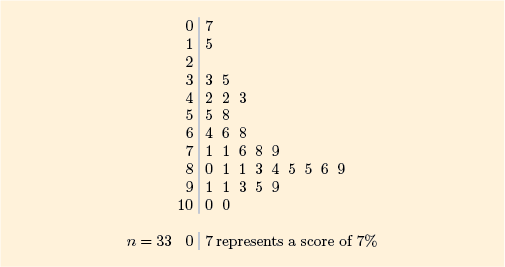Science, Maths & Technology

### Become an OU studentStart this free course now. Just create an account and sign in. Enrol and complete the course for a free statement of participation or digital badge if available.

# Exercises on Section 1

The following exercises provide extra practice on the topics covered in Section 1.

## Exercise 1 Finding medians

For each of the following batches of data, find the median of the batch. (We shall also use these batches of data in some of the exercises in Section 3.)

(a) Percentage scores in arithmetic obtained by 33 school students.Figure 8

### Discussion

For the arithmetic scores, the position of the median is , so the median is 79%.

(b) Prices of 26 digital televisions with 22- to 26-inch LED screens, quoted online by a large department store in February 2012. The prices have been rounded to the nearest pound (£).

 170 180 190 200 220 229 230 230 230 230 250 269 269 270 279 299 300 300 315 320 349 350 400 429 649 699

### Discussion

For the television prices, the position of the median is , so the median is halfway between and . Thus, the median is

## Exercise 2 Finding means

Calculate the mean for each of the batches in Exercise 1.

### Discussion

For the batch of arithmetic scores in part (a) of Exercise 1, the sum of the 33 values is 2326 and

Therefore, the mean is 70.5%. (The original data are given to the nearest whole number, so the mean is rounded to one decimal place.)

For the batch of television prices in part (b) of Exercise 1, the sum of the 26 values is 7856 and

Therefore, the mean is £302.2.

## Exercise 3 The effect of removing values on the median and mean

In the data on prices for small televisions in Activity 1 (Subsection 1.2), the three highest-priced televisions were considerably more expensive than all the others (which all cost under £200). Suppose that in fact these prices had been for a different, larger type of television that should not have been in the batch. (In fact that is not the case – but this is only an exercise!) Leave these three prices out of the batch and calculate the median and the mean of the remaining prices.

How do these values compare with the original median (150) and mean (162)? What does this comparison demonstrate about how resistant the median and mean are?

### Discussion

For the median, there are now 17 prices left in the batch, so the median is at position . It is therefore 150.

The sum of the remaining 17 values is 2480, so the mean is

In this case, removing the three highest prices has not changed the median at all, but it has reduced the mean considerably. This illustrates that the median is a more resistant measure than the mean.